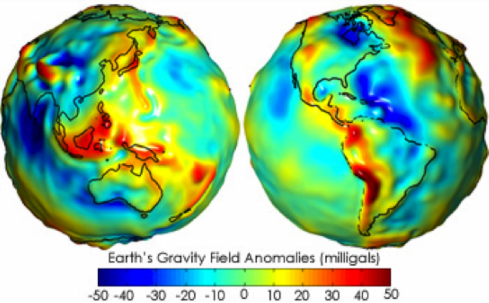Forces and Motion

Equation

Fg = Gm1m2———r2

1.10

Gravity

Universal Law of GravityDiagram from Pennsylvania Dept. of EducationThe force of gravity was first determined by Sir Isaac. He proposed that the force of gravity extends beyond the Earth and could even be responsible for holding the Moon in orbit. His Universal Law of Gravity states that every object in the universe attracts every other object with a force directed along the line of centers for the two objects that is proportional to the product of their masses and inversely proportional to the square of the separations between the two objects:

Fg = Gm1m2———r2Here m1 and m2 are two masses such as the Earth and the Moon, r is the distance between them, and G is the universal gravitational constant = 6.67×10-11  Nm2/kg2

The force acting on each mass is equal: F1 = F2

These units are unfamiliar. N stands for a Newton, which is the unit of force, being 1 kg*(m/s2), so this constant is a Newton meter squared, for each kilogram squared.

This equation applies just as well on Earth as it does for distant galaxies, it is universal.Try It!

The Sun and the Earth are separated by approximately 150 million km. The Sun has a mass of 2 x 1030 kg. The Earth’s mass is 6 x 1024 kg. What is the force of gravity between the sun and the Earth? Remember to convert distances to meters.

N

Planetary GravityWhile gravity is nearly the same across Earth it actually does have small variations that relate to the large scale geology of the Earth. NASA has launched a number of spacecraft missions to accurately measure gravity for Earth, the Moon and other planets to investigate the geology below ground that we can’t otherwise see. This map shows gravity anomalies – differences from the average – from the GRACE satellites (Gravity Recovery And Climate Experiment). The areas where gravity is strongest (shown in red) tend to be where plate tectonics activity is also strongest, for example, along the Andes Mountains.